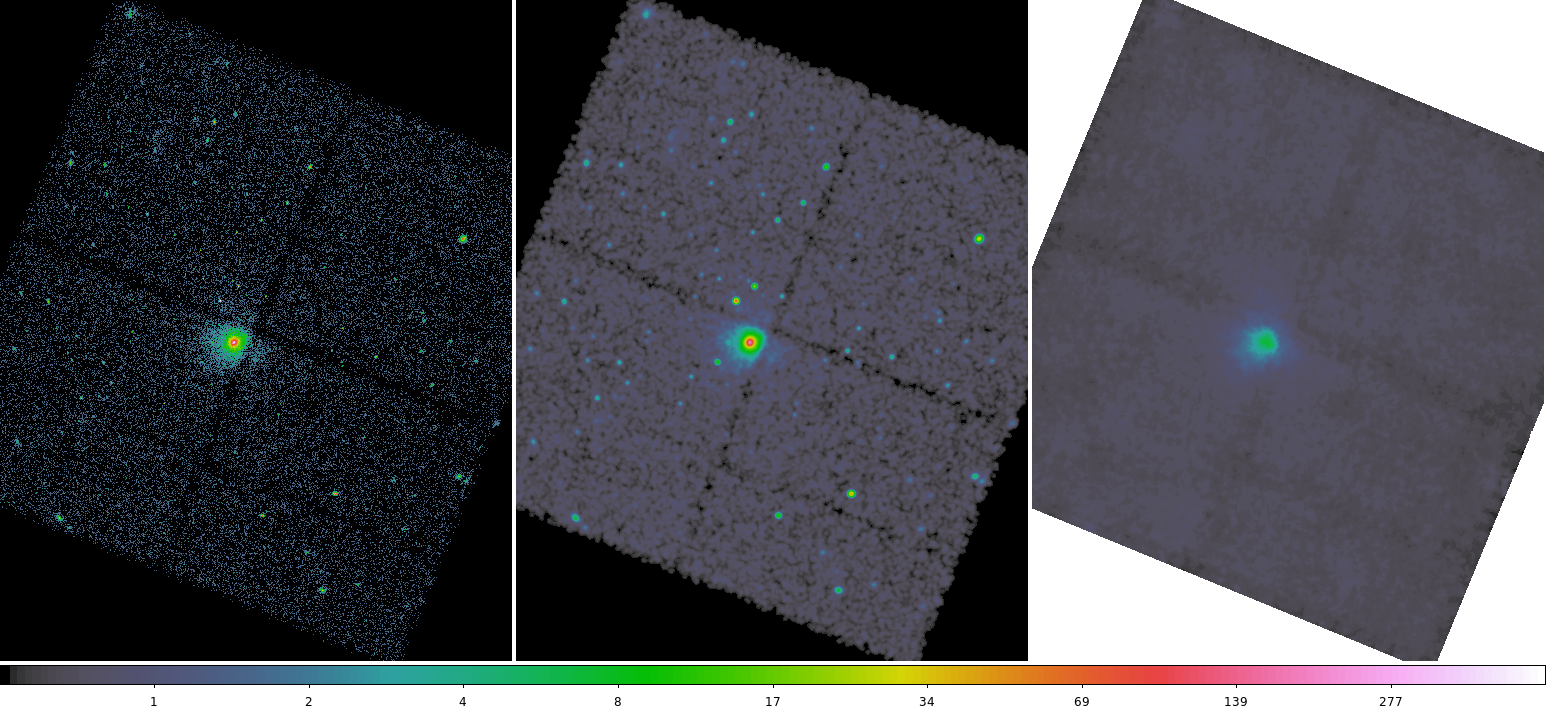# An Estimated Background Image## Overview

Last Update: 5 Feb 2010 - reviewed for CIAO 4.2: no changes

Synopsis:

This thread shows one method for making an estimated background image for a dataset. The resulting image can be used with any data analysis tool that takes a background image, e.g. wavdetect or dmextract.

you need to create a background image for an ACIS or HRC imaging observation. This method is suited to fields which contain a few point sources; it will not work well with very large extended objects.

## Get Started

Sample ObsID used: 578 (ACIS-S, 3C 295)

File types needed: evt2; fov1; asol1 (to run skyfov only)

The sky field of view file (fov1.fits) is part of the standard data distribution for observations processed with ASCDSVER 6.9.0 or later. If your data has an earlier software version, as ObsID 578 does, use the tool skyfov to create the file:

```unix% dmkeypar acisf00578N002_evt2.fits ASCDSVER echo+
6.1.0

```

In many cases, there will be more than one aspect solution file (pcad_asol1.fits) for an observation. All the files must be input, either as a list or as a stack. Here we use:

```unix% cat pcad_asol1.lis
```

The contents of the parameter file may be checked using plist skyfov.

## Make an Image of the Chip

The event data is binned into an image, using the field of view file to select the desired chips. For this example, ACIS-S3 (ccd_id=7) is selected:

```unix% dmcopy "acisf00578N002_evt2.fits[ccd_id=7,sky=region(578_fov1.fits[ccd_id=7])][bin sky=2]" 578_img.fits
```

## Convolve the Image

The integer image is first convolved with a 3-sigma Gaussian by aconvolve to smooth out the zero-value pixels. This will produce better results from the median-value filter applied in the next step.

```unix% punlearn aconvolve
unix% pset aconvolve infile=578_img.fits
unix% pset aconvolve outfile=578_convolved_img.fits
unix% pset aconvolve kernelspec="lib:gaus(2,5,1,3,3)"
unix% pset aconvolve meth=fft

unix% aconvolve
Input file name (578_img.fits):
Kernel specification (lib:gaus(2,5,1,3,3)):
Output file name (578_convolved_img.fits):
```

The contents of the parameter file may be checked using plist aconvolve.

## Filter the Image

A "median ring" filter is applied to the file. The median is the middle value from the array of pixel values. Note that dmimgfilt uses a slightly non-standard median: if the number of points is even, then the lower value is used rather than the mean of the two middle values.

The radii of the annulus should chosen be such that the region is big enough to exclude any structure you want removed (e.g. point sources) and small enough so that you're not sampling data too far away from the current pixel. In this example, an inner radius of 20 pixels and outer radius of 22 pixels are used.

```unix% punlearn dmimgfilt
unix% pset dmimgfilt infile=578_convolved_img.fits
unix% pset dmimgfilt outfile=578_bg.fits
unix% pset dmimgfilt function=median

unix% dmimgfilt
Input image file name (578_convolved_img.fits):
Output file name (578_bg.fits):
Filter function (min|max|mean|median|...|rms) (median):
```

The contents of the parameter file may be checked using plist dmimgfilt.

The file 578_bg.fits can be used as input to wavdetect, dmextract, or any data analysis tool that uses a background image. The final background image is shown in Figure 1.

### Figure 1: Estimated background image compared to source### Figure 1: Estimated background image compared to source

The background image (578_bg.fits) is in the left frame. The right frame is the source image (578_img.fits) created in the Make an Image of the Chip section.

```

infile = acisf00578N002_evt2.fits Name of input file, event list, image fits, or obi par file
outfile = 578_fov1.fits     Name of output file
(logfile = STDOUT)          Name of log file
(kernel = FITS)            Output file format, ASCII or FITS
(aspect = )                Aspect file or a stack of aspect file list
(mskfile = )                Mask file to retain active windows of chips
(geompar = geom)            Pixlib geometry parameter file
(clobber = no)              Overwrite existing outfile (yes|no)?
(verbose = 0)               Verbosity level (0 = no display)
(mode = ql)
```

```

infile = 578_img.fits     Input file name
outfile = 578_convolved_img.fits Output file name
kernelspec = lib:gaus(2,5,1,3,3) Kernel specification
(writekernel = no)              Output kernel
(kernelfile = ./.)             Output kernel file name
(writefft = no)              Write fft outputs
(fftroot = ./.)             Root name for FFT files
(method = fft)             Convolution method
(edges = wrap)            Edge treatment
(const = 0)               Constant value to use at edges with edges=constant
(center = no)              Center FFT output
(normkernel = area)            Normalize the kernel
(clobber = no)              Clobber existing output
(verbose = 0)               Debug level
(mode = ql)
```

```

infile = 578_convolved_img.fits Input image file name
outfile = 578_bg.fits      Output file name
function = median           Filter function
(numiter = 1)               Number of iterations to loop over
(verbose = 0)               Tool verbosity
(clobber = no)              Clobber existing output?
(mode = ql)
(box3 = box(0,0,3,3))    Box: 3x3
(box5 = box(0,0,5,5))    Box: 5x5
(box7 = box(0,0,7,7))    Box: 7x7
(circle3 = circle(0,0,3))   Circle: r=3
(circle5 = circle(0,0,5))   Circle: r=5
(circle7 = circle(0,0,7))   Circle: r=7
(annulus5_3 = annulus(0,0,3,5)) Annulus: o=5,i=3
(annulus7_5 = annulus(0,0,5,7)) Annulus: o=7,i=5
(annulus7_3 = annulus(0,0,3,7)) Annulus: o=7,i=3
(bann5_3 = box(0,0,5,5)-box(0,0,3,3)) Box Annulus: o=5,i=3
(bann7_3 = box(0,0,7,7)-box(0,0,3,3)) Box Annulus: o=7,i=3
(bann7_5 = box(0,0,7,7)-box(0,0,5,5)) Box Annulus: o=7,i=5
```

## History

 13 Jul 2009 New for CIAO 4.1 05 Feb 2010 reviewed for CIAO 4.2: no changes